Courses

# Test: Basic Concepts Of Differential And Integral Calculus- 2

## 40 Questions MCQ Test Business Mathematics and Logical Reasoning & Statistics | Test: Basic Concepts Of Differential And Integral Calculus- 2

Description
This mock test of Test: Basic Concepts Of Differential And Integral Calculus- 2 for CA Foundation helps you for every CA Foundation entrance exam. This contains 40 Multiple Choice Questions for CA Foundation Test: Basic Concepts Of Differential And Integral Calculus- 2 (mcq) to study with solutions a complete question bank. The solved questions answers in this Test: Basic Concepts Of Differential And Integral Calculus- 2 quiz give you a good mix of easy questions and tough questions. CA Foundation students definitely take this Test: Basic Concepts Of Differential And Integral Calculus- 2 exercise for a better result in the exam. You can find other Test: Basic Concepts Of Differential And Integral Calculus- 2 extra questions, long questions & short questions for CA Foundation on EduRev as well by searching above.
QUESTION: 1

Solution:
QUESTION: 2

Solution:
QUESTION: 3

### The derivative of (x2–1)/x is

Solution:
QUESTION: 4

The differential coefficients of (x2 +1)/x is

Solution:
QUESTION: 5

If y =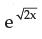then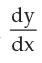is equal to _____.

Solution:
QUESTION: 6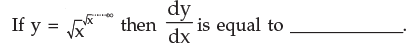Solution:
QUESTION: 7

If x = (1 – t2 )/(1 + t2) y = 2t/(1 + t2) then dy/dx at t =1 is _____________.

Solution:
QUESTION: 8

f(x) = x2/ex then f ’(1) is equal to _____________.

Solution:
QUESTION: 9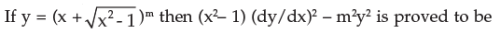Solution:
QUESTION: 10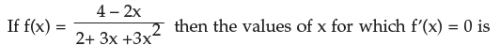Solution:
QUESTION: 11

Evaluate ∫ 5x2 dx  and the answer will be

Solution:
QUESTION: 12

Integration of 3 – 2x – x4 will become

Solution:
QUESTION: 13

Given f(x) = 4x3 + 3x2 – 2x + 5, ∫ f(x) dx   is

Solution:
QUESTION: 14

Evaluate ∫ (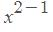) dx . The value is

Solution:
QUESTION: 15

∫ (1 - 3x) (1 + x) dx is equal to

Solution:
QUESTION: 16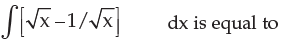Solution:
QUESTION: 17

The integral of px3 + qx2 + rk + w/x is equal to

Solution:
QUESTION: 18

Use method of substitution to integrate the function f(x) = (4x + 5)6 and the answer is

Solution:
QUESTION: 19

Use method of substitution to evaluate ∫ x (x2 + 4)5dx and the answer is

Solution:
QUESTION: 20

Integrate (x + a)n and the result will be

Solution:
QUESTION: 21

∫ 8x2/ (x3 + 2)3 dx is equal to

Solution:
QUESTION: 22

Using method of partial fraction find the integration of f(x) when f(x) = 1/x2 – a2 and the answer is

Solution:
QUESTION: 23

Use integration by parts to evaluate ∫ x2 e3x dx and the answer is

Solution:
QUESTION: 24

​∫ logx dx is equal to

Solution:
QUESTION: 25

∫ x ex dx is

Solution:
QUESTION: 26

∫ (logx)2 dx and the result is

Solution:
QUESTION: 27

Using method of partial fraction to evaluate ∫ (x + 5) dx/(x + 1) (x + 2)2 and the final answer becomes

Solution:
QUESTION: 28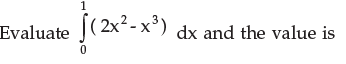Solution:
QUESTION: 29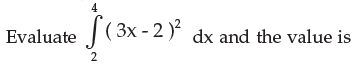Solution:
QUESTION: 30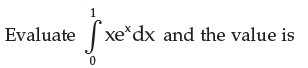Solution:
QUESTION: 31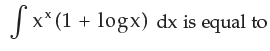Solution:

Let x
x = t. Then,d(xx) = dt
d(exlogx) = dt
exlogx (logx+1)dx = dt
xx(1+logx)dx=dt
Therefore, I = ∫xx(1+logx) dx
I= ∫dt = t+C = x+ C

QUESTION: 32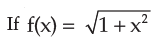then ∫ f(x)dx is

Solution:
QUESTION: 33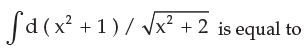Solution: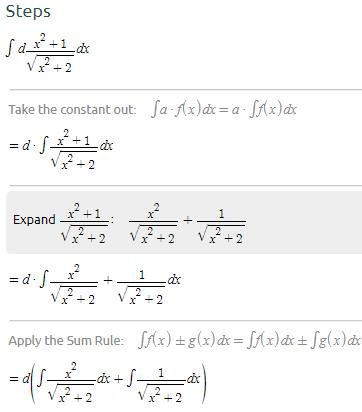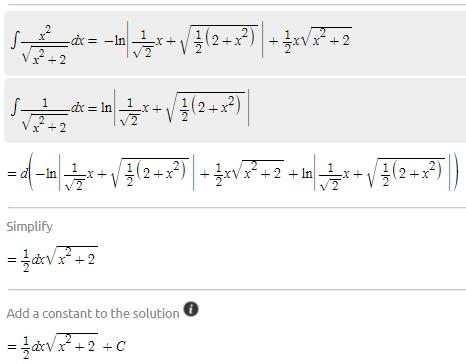QUESTION: 34

∫ (ex – e–x)2 (ex – e–x) dx is

Solution:
QUESTION: 35

a0 [ f(x) + f(–x) ] dx is equal to

Solution:
QUESTION: 36

∫ xex/(x + 1)2 dx is equal to

Solution:
QUESTION: 37

∫ (x4 + 3/x ) dx is equal to

Solution:
QUESTION: 38

Evaluate the integral ∫ (1 − x)3/ x dx and the answer is equal to

Solution:
QUESTION: 39

The equation of the curve in the form y = f(x) if the curve passes through the point (1, 0) and f’(x) = 2x – 1 is

Solution:
QUESTION: 40

Evaluate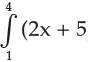dx and the value is

Solution:

Track your progress, build streaks, highlight & save important lessons and more!

### Similar Content### Related tests